Chemguide: Support for CIE A level Chemistry

```
```

Learning outcome 26: Reaction kinetics

26.1: Simple rate equations, orders of reaction and rate constants

```
```

Learning outcome 26.1.1

This statement is about some terms that you will come across in rates of reaction work - rate equation, order of reaction, rate constant, half-life of a reaction and rate-determining step.

These are terms that you will meet in the course of the rest of the statements in this section. I suggest you make a list of them for now, and tick them off as you get to them and understand them.

```
```

Learning outcome 26.1.2

This statement is about rate equations and orders of reaction and how they can be found experimentally. It also includes some terms from statement 26.1.1 - rate equation, order of reaction, rate constant. Read the relevant bits of the syllabus as you go along.

This is going to be a fairly long page!

```
```

Statement 21.1.2(a)

This statement is about the importance and use of rate equations.

First read the page orders of reaction and rate equations. Ignore the link near the bottom of the page to a further look at rate constants.

Statement 26.1.1 asks you to explain and use the term order of reaction. Using it isn't very difficult; explaining it is quite difficult to do quickly in terms of a short definition on a structured exam paper.

I would be fairly surprised if you were asked to do this, but if you were, there isn't a simple definition in words which allows you to easily distinguish between the orders with respect to individual substances and the overall order of the reaction.

Instead, I would write a general rate equation as shown on the page you have just read, and then just say that the terms "a" and "b" are known as the orders of reaction with respect to A and B, and that the overall order of the reaction equals a+b.

In other words, you explain it mainly in symbols rather than in words. I would do something similar for the term rate constant as well - write a general rate equation, and point out that the symbol k is called the rate constant.

```
```

Statement 21.1.2(b) and 21.1.2(c)

These statements are about finding orders of reaction from concentration-time graphs, initial rate experiments and half-life methods. Rate constants are dealt with separately in statement 26.1.4, and half-life in statement 26.1.3

There is no Chemguide page about this because it is covered in detail in my chemistry calculations book on pages 127 to 136, and I can't repeat it here.

All I can do is to give you a brief outline of how to do it.

```
```

Finding orders from initial rate experiments

When you do a reaction, it tends to start off quickly and then slow down as the reagents get used up. If you measure the rate at the very beginning of the reaction, you actually know exactly what the concentrations of all the reagents are at that time, because nothing has been used up yet.

If you then change the concentrations you are using, and repeat the experiment, you can relate any change in rate to what you have done to the concentrations. This is easier to see with an example.

```
```

Example 1

This is the sort of data you might be given. Remember that [A] means the concentration of A in mol dm-3.

```
```
Experiment[A]
(mol dm-3)
[B]
(mol dm-3)
initial rate
(mol dm-3 s-1)
10.100.100.0015
20.200.100.0030
30.200.200.0120
```
```

In simple cases like this, you can just look at the numbers.

When you double the concentration of A between experiments 1 and 2 (keeping [B] constant), you double the rate. The rate is proportional to [A], and so the reaction is first order with respect to A.

Between experiments 2 and 3, you keep the concentration of A constant, but double [B]. The rate goes up 4 times. So the rate is proportional to the square of the concentration of B, and the reaction is second order with respect to B.

That means that the rate equation is:

Rate = k[A][B]2

You can now go on to use this to find a value for the rate constant, k. You will find this covered in statement 26.1.4, and we will continue this calculation there.

```
```

Example 2

CIE, being CIE, are much more likely to confuse things, of course! This example is similar to one set by CIE, and you wouldn't want to meet this for the first time in an exam.

```
```
Experiment[A]
(mol dm-3)
[B]
(mol dm-3)
initial rate
(mol dm-3 s-1)
10.120.160.0014
20.120.240.0021
30.150.320.0035
```
```

You are forced to start with experiments 1 and 2, because those are the only ones where one of the concentrations is constant. This time the relationship isn't as simple as a doubling.

Find out how many times the concentration of B has increased by dividing 0.24 by 0.16. It has gone up 1.5 times.

Now do the same thing for the rate. That has gone up 1.5 times as well, and so the reaction is first order with respect to B. That's not too difficult.

The more confusing thing is working out the order with respect to A, because the concentration of B is never held constant.

Choose a pair of reactions where the concentration of B has changed in some simple way (if possible). It doesn't really matter, but you might as well make life as easy for yourself as you can.

In this example, it would make more sense to choose experiments 1 and 3 where its concentration has doubled. (If you chose 2 and 3, its concentration has gone up 1.333333(etc) times. That feels more awkward!)

If the concentration of A had stayed the same between experiments 1 and 3, the rate would have doubled because of the doubling of [B]. In other words it would have increased to 0.0028.

But, in fact, it has gone up to 0.0035. This additional increase is because of the increase in the concentration of A.

How many times has the rate increased due to A? Divide 0.0035 by 0.0028. (Remember that 0.0028 is what the rate would have been if you just doubled the concentration of B.) It has gone up 1.25 times due to the change in concentration of the A.

If you divide 0.15 by 0.12 to find out how many times the concentration of A has gone up between experiment 1 and 3, you will find that is also 1.25 times. So it is first order with respect to A as well.

That means that the rate equation is:

Rate = k[A][B]

Again you can go on from here to calculate a value for the rate constant. We'll come back to this question again in statement 26.1.4.

```
```

From half-lives

You obviously can't do this until you know about half-lives. This is covered in statement 26.1.3, and you will find examples there of working out rate constants from half-lives.

```
```

Finding orders from rate-concentration graphs

I will look at these first, because they are easier to deal with. The shape of the graph depends on whether you have a zero, first or second order reaction.

In each case, the concentration axis is labelled with the concentration of a single reagent, A. That may be because A is the only thing taking part in the reaction.

However, if there are other reactants, their concentrations will have been kept constant as you vary the concentration of A, so that the only variable is [A].

For zero order reactions

If a reaction is zero order with respect to a reactant, the rate is independent of the concentration of the reactant. That means that as you increase its concentration, the rate won't change.

If A was the only reactant, then:

Rate = k

If there were other reactants whose concentrations were being kept constant, then:

Rate = k [possible constant concentrations of other things raised to some power]

The concentration of A simply doesn't appear in the rate equation.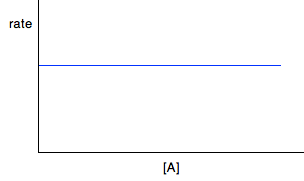For first order reactions

If a reaction is first order with respect to a reactant, the rate is proportional to its concentration. This will give you a straight line graph through the origin.

So, assuming it is first order with respect to reactant A, then if A was the only reactant:

Rate = k[A]

But there will be constant terms related to other reactants as well if A isn't the only reactant.

Rate = k [A] [possible constant concentrations of other things raised to some power]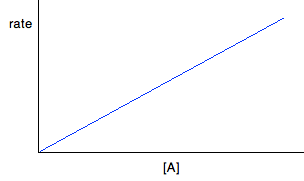For second order reactions

If a reaction is second order with respect to a reactant, the rate is proportional to the square of its concentration. This gives you a curve through the origin.

So, assuming it is second order with respect to reactant A, if A was the only reactant:

Rate = k[A]2

If A isn't the only reactant, the rate equation will look like this:

Rate = k [A]2 [possible constant concentrations of other things raised to some power]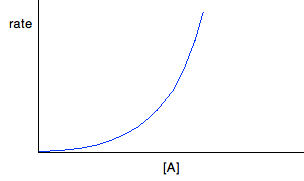But . . . you can't assume that because you have a curve, it must be second order. You will get a curve with any order other than 0 and 1. It could possibly be third order, or it might be some fractional order.

You could redraw the graph by plotting rate against [A]2, because that would give a straight line - rate is proportional to the square of the concentration.

If that doesn't give you a straight line, then there is another technique you can use - plotting a "log graph". You are unlikely to need this in a theory exam, but you might find that you have been asked to produce a log graph during a practical exercise. You will be given all the information you need. To find out more about it, you could read a small bit from this page. You will find what you want about a quarter of the way down.

```
```

Finding orders from concentration-time graphs

These are graphs taken from a single experiment in which the concentration of a reactant is measured at intervals as the reaction occurs. The order you get from these is an overall order of reaction.

It is much easier to think about these in terms of only having a single reactant, A, although I will look at a slightly more complicated case afterwards just to be on the safe side.

For zero order reactions

During any reaction, the concentrations of the reactants will obviously fall as the reaction takes place. In a zero order reaction, the rate is independent of the concentration of the reactant. That means that the concentration will fall at a steady, unchanging rate.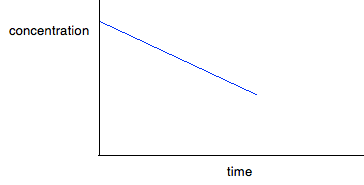For first order reactions

The curve looks like this:The curve starts falling steeply because the reaction is fastest when the concentration is at its highest. As the concentration falls, the reaction slows down, and the curve is less steep.

For second order reactions

The curve looks like this: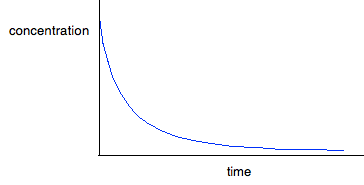If you have one of these last two curves, there is no way that you can reliably be sure which is which just by looking at it. You will meet a way of recognising the first order curve in statement 26.1.3.

```
```

A second order reaction involving A and B

If it was first order with respect to both of these, then the rate equation for this would be:

Rate = k[A][B]

If the concentrations of A and B are identical, and the reaction is 1:1 so that they always stay identical throughout the reaction, then because [A] = [B], that would give exactly the same expression as:

Rate = k[A]2

. . . and so the curve would be the same.

```
```

Statement 26.1.2(d)

This statement wants you to be able to find an initial rate using data for change of concentration against time.

The concentrations of the reactants will fall during the course of a reaction - so if you plot a graph of the concentration of one of the reactants against time, it will normally look something like this: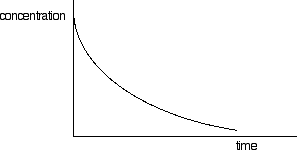The steeper the slope of the curve, the faster the reaction. You can measure the slope of the curve by drawing a tangent to it. If you want the initial rate, then you draw a tangent right at the beginning of the curve, where it hits the concentration axis.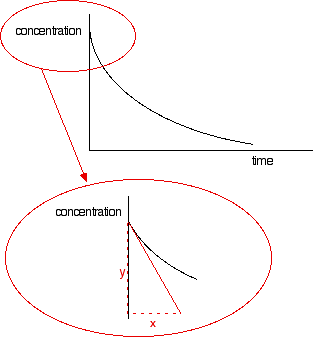The slope of the curve at that point is found by drawing a triangle and measuring the values x and y. The slope is y/x.

The units will be mol dm-3 s-1. If you have measured the time in some other units (such as minutes), these should be converted into seconds before you work out your initial rate.

Note:  Technically, this slope should be given a negative value. This shows that the concentration of the reactant is decreasing. However, in rates of reaction work, we almost always tend to ignore this.

Statement 26.1.2(e)

This statement wants you to be able to "construct a rate equation". In essence that is what all the previous statements in this section have been leading to so far.

You would find the orders with respect to the various things taking part in the reaction by looking at them one at a time. So if you had a reaction between A, B and C:

• Find the order with respect to A using one of the methods we have looked at before, keeping the concentrations of everything else constant.

• Repeat for B and C, in each case keeping the other concentrations constant.

• Slot the individual orders into the equation

rate = k[A]a[B]b[C]c

• If any of the orders are zero, leave that term out of the rate equation. Anything raised to the power zero equals 1. And if the order is 1, then you just write [A] and not [A]1.

```
```

Go to the Section 26 Menu . . .